# 5.4.4 - Univariate Statistics - Teradata Warehouse Miner

## Teradata Profiler Plug-in User Guide

Product
Release Number
5.4.4
Published
July 2017
Language
English (United States)
Last Update
2018-05-03
dita:mapPath
tjx1484331808948.ditamap
dita:ditavalPath
ft:empty
The following univariate statistics are individually selectable for analysis. By default, the following are all selected and must be selected for the graphs to be available:
• Number of values
• Minimum value
• Maximum value
• Mean value
• Standard deviation
Use Select All and Deselect All to enable or disable all options.
Statistic Description
Number of Values A count of the total number of rows (observations) with values for the specified column.

This is required for graphs.

Minimum Value The smallest value taken on by the column: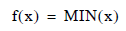This is required for graphs.

Maximum Value The largest value taken on by the column.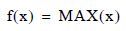This is required for graphs.

Mean Value The average value of the column.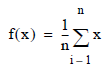Where n is the total number of rows (observations) with values for the variable x.

This is required for graphs.

Standard Deviation The standard deviation of the variable. The standard deviation is a measure of how widely values are dispersed from the average (mean) value, and is calculated on the entire population (by default).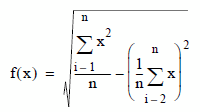Where n is the total number of rows (observations) with values for the variable x.

This is required for graphs.

Skewness Variable skewness is a characterization of the degree of asymmetry of a distribution around its mean. Positive skewness indicates a distribution with an asymmetric tail extending toward more positive values. Negative skewness indicates a distribution with an asymmetric tail extending toward more negative values.

The measures for skewness provided by Profiler is know as Fisher g statistics, related to momental skewness.

Skewness is calculated as follows, based on the entire populations: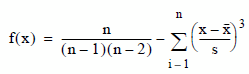Where n is the total number of rows (observations) with values for the variable xSkewness is undefined when either the standard deviation of the variable is 0, or the number of occurrences is less than 3.

Kurtosis Variable kurtosis is a characterization of the relative peakedness or flatness of a distribution compared with the normal distribution. Positive kurtosis indicates a relatively peaked distribution. Negative kurtosis indicates a relatively flat distribution.

The measures for kurtosis provided by Profiler is know as Fisher g statistics, related to momental kurtosis.

Kurtosis is calculated as follows, based on the entire populations: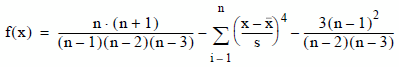Where n is the total number of rows (observations) with values for the variable xKurtosis is undefined when either the standard deviation of the variable is 0, or the number of occurrences is less than 4.

Standard Error The standard error of the variable, calculated as the standard deviation divided by the square root of the number of occurrences. Standard error is calculated as follows, based on the entire population: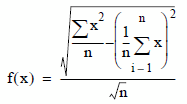Where n is the total number of rows (observations) with values for the variable x.

Coefficient of Variance The coefficient of variance of the variable, calculated as 100 times the standard deviation divided by the mean. Coefficient of variance is calculated as follows, based on the entire population: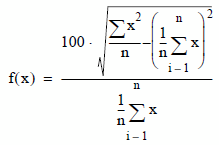Where n is the total number of rows (observations) with values for the variable x. Coefficient of variance is undefined when the average of the variable is 0.

Variance The variance of the variable, calculated as the square of the standard deviation. Variance is calculated as follows, based on the entire population: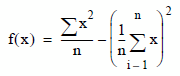Where n is the total number of rows (observations) with values for the variable x.

Sum The sum of the variable: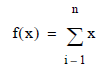Where n is the total number of occurrences of this variable.

Uncorrected Sums of Squares The uncorrected sums of squares of the variable: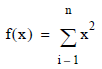Where n is the total number of occurrences of this variable.

Corrected Sums of Squares The corrected sums of squares of the variable: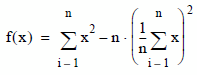Where n is the total number of occurrences of this variable.